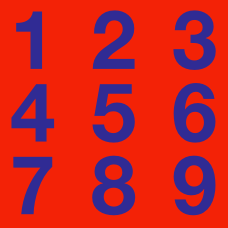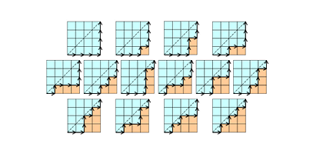Number Theory

# Number Theory Warmups: Level 5 Challenges

$2^{1}-2^{2}+2^{3}-2^{4}+2^{5}-2^{6}+2^{7} -\ldots +2^{2013}$

Let $a$ be the value of the expression above. Find the last two digits of $a.$

Given that in the 8-digit number $\overline{\mathrm{ABCDEFGH}}$,

(i) $\overline{\mathrm{ABCD}}=\overline{\mathrm{EFGH}}$
(ii) The numbers $\overline{\mathrm{ABCDEFGH}}$ and $\overline{\mathrm{ABCD0EFGH}}$ are both divisible by $11$.
Let the sum of all possible values of $\overline{\mathrm{ABCDEFGH}}$ be $N$.

Find the digit sum of $N$.

Details and assumptions:-

• $\overline{\mathrm{ABC}}$ means the number in decimal representation with digits $A,B,C$ i.e. $\overline{\mathrm{ABC}} = \mathrm{100A+10B+C}$
• The letters $A$ to $H$ do not necessarily stand for distinct digits.
• In the second number, the digit $0$ is added in the middle of the 8-digit number, which makes it a 9-digit number.
• Digit sum is sum of all digits in decimal representation, digit sum of $12023$ is $1+2+0+2+3=8$
• 00123 is not a 5-digit number.

Consider the sequence $50 + n^2$ for positive integer $n$:

$51, 54, 59, 66, 75, \ldots$

If we take the greatest common divisor of 2 consecutive terms, we obtain

$3, 1, 1, 3, \ldots$

What is the sum of all distinct elements in the second series?If we write out the decimal expansion of the smaller root of $f(x)=1000000x^2-1000000x+1$, we get the following decimal: $0.000001 \quad 000001\quad 000002\quad 000005 \quad 000014 \quad \ldots$ which we notice as the first 5 Catalan numbers. How many distinct Catalan numbers occur in a row before this pattern stops?

###### Image Credit: Wikimedia Dmharvey.

$\large \frac1a+\frac1b+\frac1c= \frac1{42}$

Let $a\leq b \leq c$ be positive integers that satisfy the equation above. Find the maximum possible value of $c$.

×